# arcto()

## Syntax

```arcto(x, y, ccw=False, close=False)
```

Draw a semicircular arc from the current pen location to the destination coordinates

```arcto(cx, cy, x, y, radius, close=False)
```

Draw a circular arc within the angle created by the current point, a control point, and the destination point

## Description

Draws a circular arc between the current point in the path and a destination point. When called with a single pair of x/y arguments, a full semicircle will connect the points. The `ccw` argument can be set to `True` to draw a mirror image of the arc.

To draw more complex arcs, include an additional x/y point to specify where the arc should ‘peak’ between the origin and destination points. An arc will be drawn at the corner of the triangle formed by the current point in the path, the control handle, and the destination point. Be sure to specify a `radius` that is small enough to fit within that angle.

If the `close` argument is set to `True`, a lineto() command will be synthesized connecting the end of the arc to the origin point of the active subpath. The arcto() command must be called inside a bezier() context (or between beginpath() and endpath()).

## Example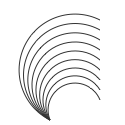```for i in range(9):
with bezier(50,120, stroke=0.2, fill=None):
arcto(100, 100-i*10)
```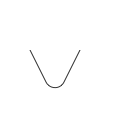```with bezier(30, 50, stroke=0.2, fill=None):
arcto(55,100, 80,50, 10)
```

# bezier()

## Syntax

```bezier(points=[], smooth=False, **opts)
```

Draw a path based on a list of Point or Curve objects (or x/y tuples)

```bezier(path, **opts)
```

Draw the provided Bezier object using the current graphics state

```with bezier(x=0, y=0, **opts) as path:
... # drawing commands like moveto(), lineto(), arcto(), or curveto()
```

Draw a path based on a starting point and a sequence of ‘to’ commands

## Description

Draws custom shapes that you define point-by-point. When called with a list as the first argument, the path will be constructed by drawing lines between the points specified in the list’s elements.

If the list contains more than three Points (or `x`/`y` tuples), the optional `smooth` argument controls how line-segments are stitched together. By default straight lines will be used, but if `smooth=True` the control handles will be positioned to minimize sharpness at the vertices.

## Context Manager

When called as part of a `with` statement, the optional `x` & `y` parameters set the location of the first point (origin) in the path. When not supplied in the call to bezier(), a starting location for the path should be given inside the indented block using the moveto() command.

Inside the `with` block, a series of other drawing commands usually follows. You can call any of the ‘to’ commands (moveto(), lineto(), etc.) as well as any of the shape-drawing primitives (arc(), poly(), line(), and friends).

The path will be drawn after the last command in the indented block unless you set the `plot` argument to `False`. In these cases, you can plot() the path later on using the variable ‘captured’ in the `as` clause of the `with` statement. Note that the captured path-variable continues to be valid even outside of the indented-block.

## Optional Parameters

`plot`
By default, paths are drawn as soon as the `with` block completes (or immediately if a `points` list was provided). But when called with `plot=False`, a reference to the path will be returned but will not be drawn. You can manually add it to the canvas later on by passing the reference to the plot() command.

`close`
If `True`, connect the final point in the path back to the starting position after the final drawing command. If omitted, it defaults to `False` (or inherits the value from the last call to autoclosepath()).

`fill` / `stroke` / `nib`
Values passed to these parameters will override the state set in previous calls to the corrseponding fill(), stroke(), and pen() commands. If omitted, the path will inherit values from the graphics state when drawn.

`cap`
Set the shape of the path’s endpoints to `BUTT`, `SQUARE` or `ROUND`. If omitted, the style defaults to `BUTT` (or inherits the value from the last call to pen() that included a `cap` parameter).

`join`
Set the shape of the path’s vertices to `MITER`, `BEVEL` or `ROUND`. If omitted, the style defaults to `MITER` (or inherits the value from the last call to pen() that included a `join` parameter).

## Examples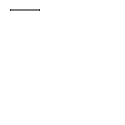```# define a path inside of a 'with' block
with bezier(10, 10, stroke=0.2) as path:
lineto(40, 10)
```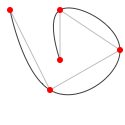```# define a list of x,y points for the path
points = [(10, 10), (50, 90), (120, 50), (60, 10), (60, 60)]

# draw the path twice; once with straight lines in light grey
# and again with smoothed lines in dark grey
nofill()
bezier(points, stroke=0.75)
bezier(points, stroke=0.25, smooth=True)

# draw red dots at the point coordinates
for x, y in points:
```

# curveto()

## Syntax

```curveto(h1x, h1y, h2x, h2y, x, y, close=False)
```

## Description

Draws a curve between the current point in the path and a new ‘destination’ point. The pair of positional args, `x` and `y`, set the location of the destination point. The first two pairs of arguments set the location of the two ‘control points’ of the curve. These control points (or handles in Illustrator nomenclature) define the edge and slant of the curve.

If the `close` argument is set to `True`, a lineto() command will be synthesized connecting the end of the curve to the origin point of the active subpath.

The curveto() command must be called inside a bezier() context (or between beginpath() and endpath()).

## Example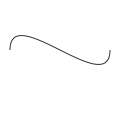```nofill()
stroke(0.2)
with bezier(10,50) as path:
curveto(10,0, 110,100, 110,50)
```
or
```nofill()
stroke(0.2)
autoclosepath(False)
beginpath(10, 50)
curveto(10,0, 110,100, 110,50)
path = endpath()
```

# lineto()

## Syntax

```lineto(x, y, close=False)
```

## Description

Draws a line between the current point in the path and a new destination point (set by the `x` and `y` parameters). If the `close` argument is set to `True`, a lineto() command will be synthesized connecting the end of the line-segment to the origin point of the active subpath.

The lineto() command must be called inside a bezier() context (or between beginpath() and endpath()).

## Example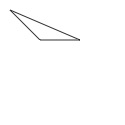```nofill()
with bezier(10, 10, stroke=0.2) as path:
lineto(40, 40)
lineto(80, 40, close=True)
```
or
```nofill()
stroke(0.2)
beginpath(10, 10)
lineto(40, 40)
lineto(80, 40)
endpath()
```

# moveto()

## Syntax

```moveto(x, y)
```

## Description

Moves the ‘current’ point of the path to a new location (x,y) without drawing a line from the previous point. This is conceptually similar to lifting your pen from the page and putting it down somewhere else before continuing to draw.

The moveto() command must be called inside a bezier() context (or between beginpath() and endpath()).

## Example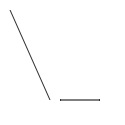```with bezier(10, 10, stroke=0.2) as path:
lineto(50, 100)
moveto(60, 100)
lineto(100, 100)
```
or
```stroke(0.2)
beginpath(10, 10)
lineto(50, 100)
moveto(60, 100)
lineto(100, 100)
endpath()
```

# Bezier

## Syntax

```Bezier()
```

## Description

Every ‘shape’ you create in PlotDevice (see for example, rect(), oval(), bezier(), textpath()) is a Bezier object. This object has a number of properties to manipulate indivdual points in the path and can be passed to the bezier() command to draw it on the canvas.

## Properties

```path.bounds
path.length
path.contours
path[i] # list elements are Curve objects
```

## Methods

```path.moveto(x, y)
path.lineto(x, y)
path.curveto(h1x, h1y, h2x, h2y, x, y)
path.closepath()
path.rect(x, y, width, height)
path.oval(x, y, width, height)
```

Adding line segments and shapes to the path

```path.contains(x, y)
```

Hit-testing

```path.point(t)
path.points(amount=100)
path.segmentlengths(relative=False, n=10)
```

Point interpolation (accessing and mutating coordinates within the Curve objects)

```path.intersects(pth)
path.intersect(pth, flatness=0.6)
path.union(pth, flatness=0.6)
path.difference(pth, flatness=0.6)
```

Boolean logical operators

```path.fit(x=None, y=None, width=None, height=None, stretch=False)
```

Clamping the path to fit based on optional constraints

`path.copy()`

See the tutorial on paths to learn about all the methods.

With the segmentlengths() method you can define the math precision for point() and points() by making n a higher value (usually 10 is fine).

When you loop over a path it returns a list of Curve objects.

# Context

## Syntax

```Context()
```

## Description

An object wrapping a canvas and all the PlotDevice commands that operate on it. Context objects are primarily useful outside of the app—when you’re writing a plain python script that imports the `plotdevice` module and uses the export() command to generate output.

## Properties

```ctx.canvas
ctx.WIDTH
ctx.HEIGHT
```

## Methods

```ctx.size()
ctx.background()
ctx.fill()
ctx.stroke()
ctx.export()
# ... and all the other commands in the PlotDevice API
```

## Example

```#/usr/bin/env python3
from plotdevice import Context

ctx = Context()
ctx.size(400, 300)
ctx.background('black', 'white', angle=0)
ctx.fill('black', 'white', angle=180)
ctx.rect(100,100, 200,100)
ctx.export('output@2x.png')
```

Note that you would run this script from the command line with the ordinary `python3` command—not the `plotdevice` command line tool.

# Curve

## Syntax

```Curve()
```

## Description

Each element of a Bezier is a Curve object representing a PostScript-style drawing command. This object has a number of properties that describe the coordinates and handles of the path element.

See the tutorial on paths to learn about how Curve and Bezier objects interact.

## Properties

```curve.cmd
curve.x
curve.y
curve.ctrl1.x
curve.ctrl1.y
curve.ctrl2.x
curve.ctrl2.y
```

The `cmd` property describes the type of line-segment this Curve belongs to. Its value can be `MOVETO`, `LINETO`, `CURVETO` or `CLOSE`. The `x` & `y` properties will be defined for all `cmd` types except for `CLOSE`. The `ctrl1` & `ctrl2` properties are only relevant if `cmd` is `CURVETO` (in which case, each property is a Point object).

## Methods

```curve.angle(point)
curve.distance(point)
curve.coordinates(distance, angle)
curve.reflect(point, d=1.0, a=180)
```

These geometry helper-methods behave the same as those supported by the Point object. They implicitly use the Curve’s `x` & `y` properties. Be careful when blindly iterating through Curves since any with a `cmd` of `CLOSE` will have (0,0) coordinates (which might give you unexpected results).

# Image

## Description

The image() command in PlotDevice returns an Image object representing a bitmap or vector image file. If you call image() with a `plot=False` argument, you can make copies of the image and position or plot() the object manually.

## Methods

```img.draw()
img.copy()
```

## Properties

```img.x
img.y
img.width
img.height
img.frame  # position & maximal size defined when creating the Image
img.bounds # position & size of the scaled image (fitted to the frame)
img.size   # the (unscaled) dimensions of the source image
```

# autoclosepath()

## Syntax

```autoclosepath(close=True)
```

## Description

Defines whether custom shapes created between beginpath() and endpath() are automatically closed by connecting the last point and the first point in the path with a line. It takes a single parameter of `True` or `False`. All shapes created with beginpath() following this command will adhere to the setting.

## PlotDevice Equivalent

The new bezier() command takes a keyword argument called `close` which, when set to `True` will close the path at the end of a `with` block. The lineto(), cuveto(), and arcto() commands will also accept a `close` argument allowing you to close the subpath after adding a line segment.

# beginpath()

## Syntax

```beginpath(x=None, y=None)
```

## Description

Using the beginpath() and endpath() commands allows creating custom shapes. The two parameters of beginpath() set the location of the first point (origin) in the path. These parameters are optional. When not supplied, a starting location for the path should be given with the moveto() command.

After calling the beginpath() command, a series of other path commands usually follows (for example moveto(), lineto(), or curveto()). Finally, the endpath() command draws the path to the screen.

## PlotDevice Equivalent

The beginpath() command has been subsumed into the new bezier() command. Rather than calling beginpath() and endpath() to construct beziers, you can call bezier() as part of a `with` statement.

## Example```stroke(0.2)
beginpath(10, 10)
lineto(40, 10)
endpath()
```

# drawpath()

## Syntax

```drawpath(path)
```

## Description

Draws a Bezier path to the screen. The path is a series of lines and curves defined between beginpath() and endpath(). Normally, endpath() draws the path to the screen, but this can be suppressed by calling it with `plot=False`. The path can then be assigned to a variable, and this variable used as a parameter for drawpath().

Note: if you have one path that you want to draw multiple times with drawpath(), for example each with its own rotation and position, you need to supply a copy:

```drawpath(path.copy())
```

## PlotDevice Equivalent

The new plot() command will draw Bezier objects (as well as images and text) to the canvas. You can also pass a Bezier object to the bezier() command and it will draw a new copy of it (inheriting the canvas’s current graphics state in the process).

## Example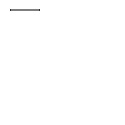```stroke(0.2)
beginpath(10, 10)
lineto(40, 10)
p = endpath(plot=False)
drawpath(p)
```

# endpath()

## Syntax

```endpath(draw=True)
```

## Description

The endpath() command is the companion to beginpath() and may only be called after the latter. When endpath() is called, the path (defined by commands between beginpath() and endpath()) is drawn to the screen. If called with `draw=False`, the path is not drawn to the screen but can be assigned to a variable. Later on, the variable can be drawn with the drawpath() command.

## Returns

a Bezier object containing the constructed path

## PlotDevice Equivalent

The endpath() command has been subsumed into the new bezier() command. Rather than calling beginpath() and endpath() to construct beziers, you can call bezier() as part of a `with` statement.

## Example```stroke(0.2)
beginpath(10, 10)
lineto(40, 10)
p = endpath(plot=False)
drawpath(p)
```

# findpath()

## Syntax

```findpath(list, curvature=1.0)
```

## Description

Constructs a fluid path from a list of coordinates. Each element in the list is a 2-tuple defining the x-coordinate and the y-coordinate. If the list defines more than three points, the curvature parameter offers some control on how separate segments are stitched together: from straight lines (0.0) to smooth curves (1.0).

## Returns

a Bezier object containing the constructed path

## PlotDevice Equivalent

The findpath() command has been subsumed into the new bezier() command, though note that it gives you less control over curvature. The boolean `smooth` argument to bezier() is equivalent to calling findpath() with a curvature of 1.0 or 0.

## Example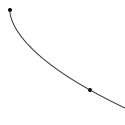```points = [(10, 10), (90, 90), (350, 200)]
for x, y in points:
oval(x-2, y-2, 4, 4)

nofill()
stroke(0.2)
autoclosepath(False)
path = findpath(points)
drawpath(path)
```